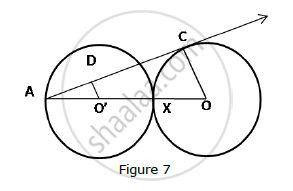# In Fig. 7, two equal circles, with centres O and O’, touch each other at X. OO’ produced meets the circle with centre O’ at A. - Mathematics

In Fig. 7, two equal circles, with centres O and O’, touch each other at X. OO’ produced meets the circle with centre O’ at A. AC is tangent to the circle with centre O, at the point C. O’D is perpendicular to AC. Find the value of (DO')/(CO')#### Solution

AO’ = O’X = XO = OC …..(Since the two circles are equal.)

So, OA = AO’ + O’X + XO …..(A-O’-X-O)

∴ OA = 3O’A

In ΔAO'D and ΔAOC,

∠DAO'= ∠CAO ....(Common angle)

∴ ΔADO' ~ ΔACO ....(By AA test of similarity)

:.(DO')/(CO')=(O'A)/(OA)=(O'A)/(3O'A)=1/3

Concept: Tangent to a Circle
Is there an error in this question or solution?

#### APPEARS IN

RD Sharma Class 10 Maths
Chapter 8 Circles
Q null | Page 37